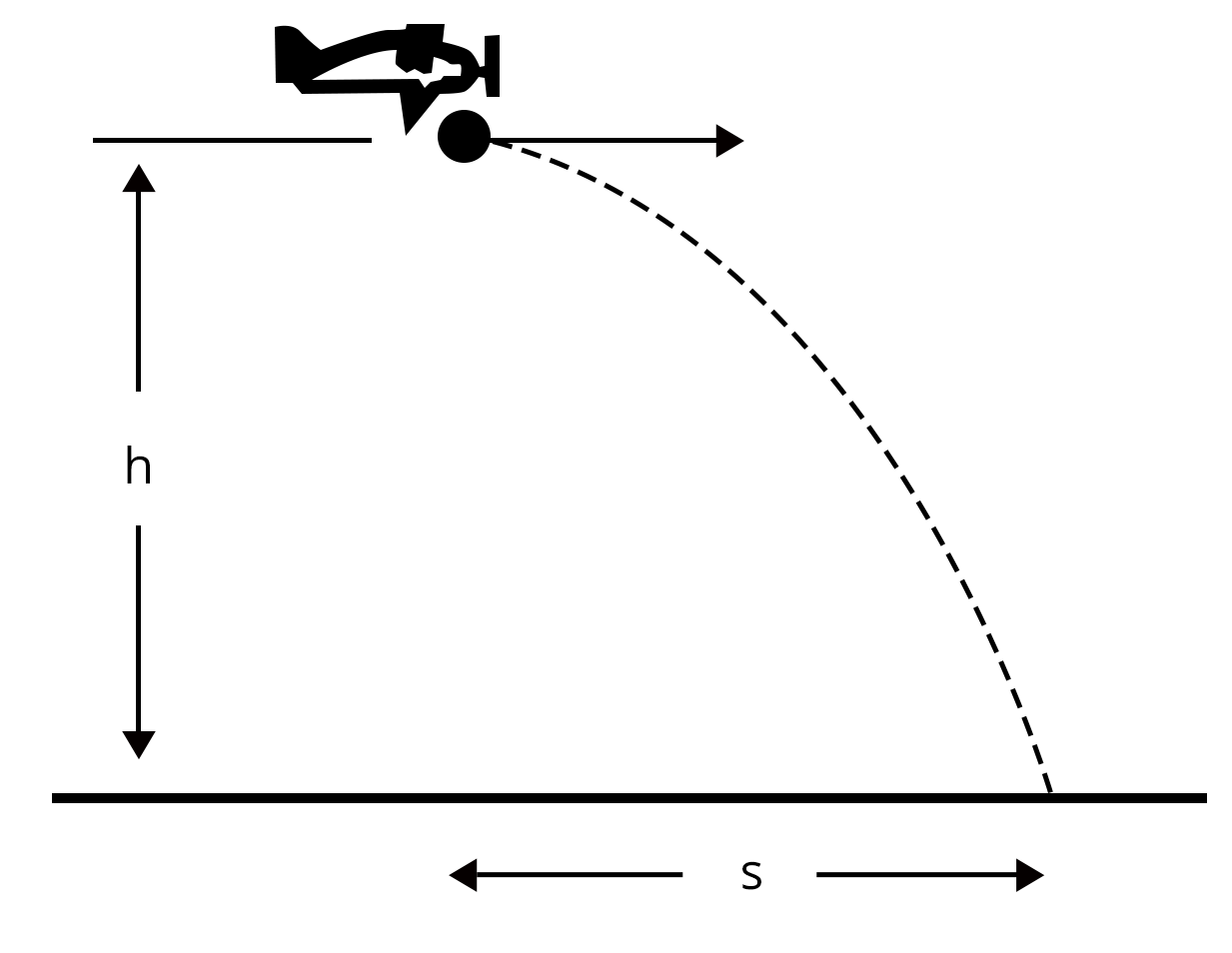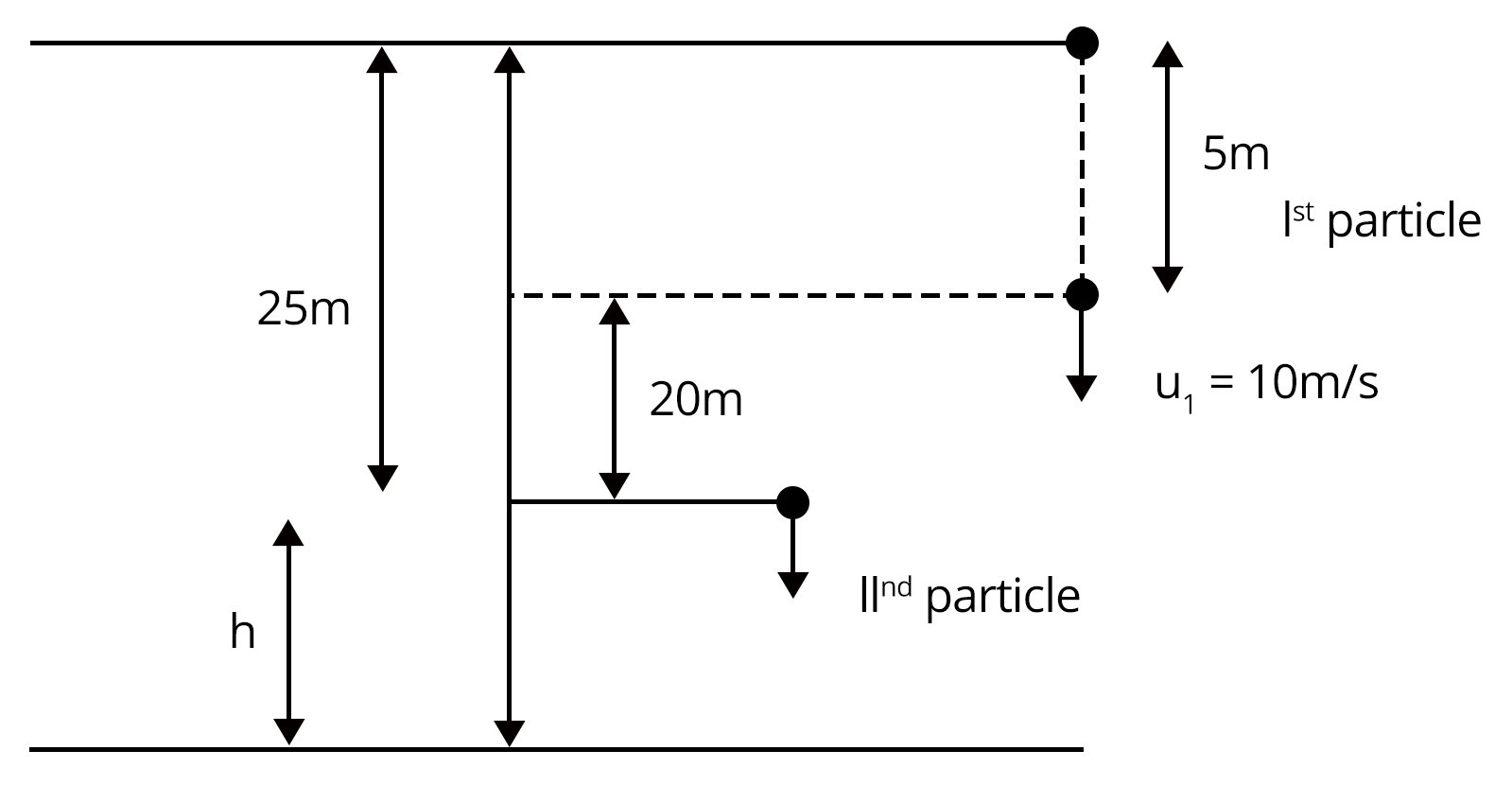Courses
Courses for Kids
Free study material
Offline Centres
More

# Kinematics Chapter - Physics JEE MainLast updated date: 01st Dec 2023
Total views: 21.3k
Views today: 1.21k## Concepts of Kinematics for JEE Main Physics

Kinematics is an important topic for JEE exams. It mainly consists of one-dimensional and two-dimensional motion, and the questions asked in the examination are based on their applications. Compared to other concepts, this section will become easier with adequate practice and conceptual understanding.

In kinematics, first, we will learn about motion along a straight line and discuss different physical quantities like distance, displacement, velocity, etc., and their formulas. Different types of motions are also discussed in this section. Problems related to the three kinematical equations of motion for uniformly accelerated motion are very important. We will also see how we can draw a conclusion about the motion by evaluating and finding required quantities from various graphs.

Coming to motion along a plane, properties of vectors and the law of vector addition like triangular law and parallelogram law of vector additions, scalar and vector product of vectors are important. The problems with projectile motion on a horizontal and inclined plane are frequently asked in JEE exam.

Let us see what is kinematics and the important concepts and formulas needed to score a full marks for this topic in JEE Main with the help of some solved kinematics examples.

## JEE Main Physics Chapters 2024

### Important Topics of Kinematics Chapters

• Motion in a Straight Line

• Kinematics Equations

• Motion under gravity

• Graphs for all types of motion

• Motion in a Plane

• Projectile Motion

• Resolution of Vector Rectangular Components

• Circular Motion

### JEE Main Kinematics Solved Examples

1. A balloon carrying a stone rises from rest on the ground with an acceleration of 10 m/s2 in the upward direction. After 5 sec, the stone is released and ultimately reaches the ground. Find the maximum height attained by the height.

Ans:  Here, we will take the upward direction as positive and the downward direction as negative. Given the balloon rises up from rest. Therefore,

The initial velocity of the balloon, u1 = 0 m/s

Acceleration of the ball, a = 10 m/s2

First of all, let us find the height reached by the balloon in 5 seconds before the stone is released using the equation of motion.

$s=ut+\dfrac{1}{2}at^{2}$

Substitute the value of each term in the equation to calculate the height reached by the balloon.

$S=0\times 5+\dfrac{1}{2}\times 10\times 5^{2}$

$S= 125 m$

Now, even after the stone is released, it will continue to move upward for some time because it has some velocity in the upward direction. Then, the initial velocity of the stone is equal to the final velocity of the balloon after 5 sec. So, let us calculate the final velocity of the balloon using the formula given below:

$v=u+at$

Substitute the values and calculate the final velocity.

$v=0+10\times 5$

$v= 50 m/s$

Now, calculate the distance covered by the stone to reach the maximum height where the final velocity is zero by using the formula given below. (Take acceleration due to gravity as -10 m/s2 since it is in the downward direction.)

$v^2=u^2+2as$

$0^2=50^2+2\times (-10)\times$

$20s=2500$

$s=125 m$

Therefore, the maximum height attained by the stone is the sum of the distance travelled by the balloon in 5 sec and the distance travelled by the stone after it is released, i.e, 125 m + 125 m = 250 m

Key Point: The velocity of any body thrown upward will be zero when it reaches its highest point but the acceleration will be equal to the acceleration due to gravity acting downwards.

2. An aeroplane moving at a speed of 180 km/hr drops a food packet while flying at a height of 500 m. Find the horizontal range?

Ans: We will consider the motion along with vertical and horizontal directions separately to apply equations of motion.

First, find the time taken by the packet to reach the ground considering motion along the vertical direction.The vertical displacement of the packet, Sy = h = 500 m

Initial velocity along vertical direction, uy = 0 m/s

Acceleration of the packet along vertical direction = g = 10 m/s2

$s_y=u_yt+\dfrac{1}{2}a_yt^{2}$

$S_y=u_yt+\dfrac{1}{2}a_yt^{2}$

$500 =0\times t+\dfrac{1}{2}\times 10t^{2}$

$1000=10t^{2}$

$t^2=100$

$t=10s$

Now consider the motion along horizontal direction and calculate the horizontal range using the equation of motion.

Initial velocity along the horizontal direction, ux = 180 km/hr = $180\times \dfrac{5}{18}$ = 50 m/s

Acceleration along the horizontal direction, ax = 0 m/s2

Time taken to reach the ground, t = 10s

$S_x=u_xt+\dfrac{1}{2}a_xt^{2}$

$S=50\times10+\dfrac{1}{2}\times 0\times 10^{2}$

$S_x= 500 m$

Key Point:  Whenever dealing with the problems related to the two-dimensional motion of a body under gravity, consider the motion along with vertical and horizontal directions separately to apply equations of motion.

### Previous Year Questions from JEE Exam

1. Two guns A and B can fire bullets at speeds 1 km/s and 2 km/s respectively. From a point on horizontal ground, they are fired in all possible directions. The ratio of maximum areas covered by bullets fired by two guns on the ground is… (JEE 2019)

Ans: For a projectile motion, maximum range is attained at an angle of 45o. The area covered by the bullets will be equal to the area of the circle with a radius equal to the maximum range of each bullet.

Bullets fired by gun A:

Let RA be the maximum range of the bullets fired by gun A at an angle of  45o.

The velocity of the bullets fired by gun A, uA = 1 km/s

The maximum range of the bullet from gun A is given by

$R_A=\dfrac{u_A^2\sin(2\theta)}{g}$

$R_A=\dfrac{u_A^2\sin(2\times45)}{g}$

$R_A=\dfrac{u_A^2}{g}$

Maximum area covered by the bullets from gun A is

$Area_A=\pi R_A^2$

$Area_A=\pi\left( \dfrac{u_A^4}{g^2}\right)$

Similarly,

The velocity of the bullets fired by gun B, uB = 2 km/s

The maximum range of the bullet from gun B is given by

$R_B=\dfrac{u_B^2}{g}$

Maximum area covered by the bullets from gun B is

$Area_B=\pi R_B^2$

$Area_B=\pi\left( \dfrac{u_B^4}{g^2}\right)$

Then the ratio of two area covered by the bullets fired by gun A and gun B is,

$\dfrac{Area_A}{Area_B}=\dfrac{\pi\left( \dfrac{u_A^4}{g^2}\right)}{\pi\left( \dfrac{u_B^4}{g^2}\right)}$

$\dfrac{Area_A}{Area_B}=\dfrac{u_A^4}{u_B^4}=\dfrac{1^4}{2^4}=\dfrac{1}{16}$ Therefore, the ratio of area covered by the bullets A and B is 1:16.

Key Point:  The maximum range is attained by a given projectile for a given velocity at an angle of 450

2. A stone is dropped from the top of a building. When it crosses a point 5m below the top, another stone starts to fall from a point 25m below the top, both stones reach the bottom of the building simultaneously. The height of the building is: (Take g = 10 m/s2) (JEE 2021 Feb)Ans: First, we have to  calculate the velocity of the first stone (u1) at a height 5 m below the top of the building using the formula given below.

$u_1=\sqrt {2gh}$

$u_1=\sqrt {2\times 10\times 5}=10m/s$

Now, apply the 2nd equation of motion on the first stone at a point 5 m below from the top till it reaches the ground. Then the displacement covered by the first stone is 20+h.

$20+h=u_1t+\dfrac{1}{2}gt^{2}$

$20+h=10t+\dfrac{1}{2}gt^{2}$.....(1)

For the second stone, the displacement covered to reach the ground is h and the time taken is t. Using the 2nd equation of motion,

$20+h=10t+\dfrac{1}{2}gt^{2}$.....(2)

Put equation (2) in equation (1) to calculate the time t

$20+\dfrac{1}{2}gt^{2}=10t+\dfrac{1}{2}gt^{2}$

t=2s

Now, put t=2s in equation (2) to calculate h

$h=\dfrac{1}{2}\times 10\times 2^{2}=20 m$

The total height of the building = h + 25= 20 + 25 = 45 m

Key Point:  Whenever an object is dropped or released, the initial velocity must be taken as zero.

### Practice Questions

1. A body is projected at 600 with the ground. It covers a horizontal distance of 100 m. If the same body is projected at 600 with vertical with the same velocity, what will be the new range? (Ans: 100 m)

2. A car moving with a speed of 50 km/hr, can be stopped by brakes after at least 6m. If the same car is moving at a speed of 100 km/hr, what will be the minimum stopping distance? (Ans: 24 m)

## JEE Main Physics Kinematics Study Materials

Here, you'll find a comprehensive collection of study resources for Kinematics designed to help you excel in your JEE Main preparation. These materials cover various topics, providing you with a range of valuable content to support your studies. Simply click on the links below to access the study materials of Kinematics and enhance your preparation for this challenging exam.

 JEE Main Kinematics Study Materials JEE Main Kinematics Notes JEE Main Kinematics Important Questions JEE Main Kinematics Practice Paper

## JEE Main Physics Study and Practice Materials

Explore an array of resources in the JEE Main Physics Study and Practice Materials section. Our practice materials offer a wide variety of questions, comprehensive solutions, and a realistic test experience to elevate your preparation for the JEE Main exam. These tools are indispensable for self-assessment, boosting confidence, and refining problem-solving abilities, guaranteeing your readiness for the test. Explore the links below to enrich your Physics preparation.

 JEE Main Physics Study and Practice Materials JEE  Main Physics Previous Year Question Papers JEE Main Physics Mock Test JEE Main Physics Formula JEE Main Sample Paper JEE Main Physics Difference Between

### Conclusion

The chapter on Kinematics is a vital component of JEE Main, serving as the foundation for understanding motion and its intricacies. It equips students with the tools to analyze and interpret the dynamics of objects, whether in classical mechanics or in the realm of modern physics. A profound grasp of kinematic principles not only paves the way for excelling in JEE Main but also lays the groundwork for comprehending more advanced physics concepts. The ability to unravel the mysteries of motion and translate them into mathematical equations is not just beneficial for succeeding in exams, but it also underpins innovations in engineering, technology, and scientific research.

## FAQs on Kinematics Chapter - Physics JEE Main

1. How many questions are asked from Kinematics in JEE Main?

Mostly, 1-2 questions from this chapter are asked in JEE Main every year which corresponds to around 8 marks in the JEE Main exam.

2. What is the importance of kinematics in the JEE exam?

Compared to other chapters, kinematics concepts will become easier for you once you start practicing more and more questions related to it. You can easily solve all the types of kinematics problems asked from this chapter once you learn all the topics covered in the chapter conceptually. Besides, the concepts discussed in this chapter are linked to problems from other chapters too.

### Repeaters Course for JEE 2022 - 23## Notice board

JEE News
JEE Blogs